Next: Problem Up: Physical Background Previous: Basic Equations of Hydrodynamics   Contents

# The Poisson Equation of the Self-Gravity

In this section, we will show the basic equation describing how the gravity works. First, compare the gravity and the static electric force. Consider the electric field formed by a point charge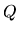at a distance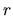from the point source as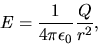(2.6)

where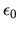is the electric permittivity of the vacuum. On the other hand, the gravitational acceleration by the point mass of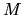at the distancefrom the point mass is written down as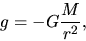(2.7)

where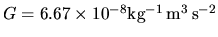is the gravitational constant. Comparing these two, replacingwithand at the same time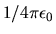to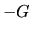these equations (2.6) and (2.7) are identical with each other.

The Gauss theorem for electrostatic field as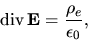(2.8)

and another expression using the electrostatic potential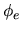as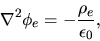(2.9)

lead to the equations for the gravity as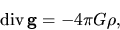(2.10)

and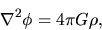(2.11)

where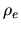and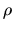represent the electric charge density and the mass density. Equation (2.11) is called the Poisson equation for the gravitational potential and describes how the potential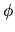is determined from the mass density distribution.

SubsectionsNext: Problem Up: Physical Background Previous: Basic Equations of Hydrodynamics   Contents
Kohji Tomisaka 2007-07-08